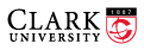Math 131, Multivariate Calculus Spring 2014 Prof. D. Joyce, BP 322, 793-7421 Department of Mathematics and Computer Science Clark University

Gauss-Weber Monument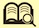Wilhelm Weber (1804–1891) and Carl Friedrich Gauss (1777–1855)
Gauss-Weber Monument in Göttingen
Date 1899, by Carl Ferdinand Hartzer

General information

• General description. Multivariate calculus uses linear algebra to extend the important concepts of single-variable calculus to higher-dimensional settings. Topics include scalar-valued and vector-valued functions, graphs, level sets, limits and continuity; partial derivatives, gradients, tangent planes, differentiability, total derivatives, directional derivatives; paths, velocity, acceleration, arclength, curvature, vector fields, divergence, curl; extrema, Hessians, Lagrange multipliers; multiple integrals, change of variables, Jacobians; line integrals, Green’s theorem; surface integrals, Stokes’ theorem, and Gauss’ theorem.

• Course prerequisite :  Math 122, 125, or 130, others by permission only.

• Web pages for related courses
Math 120, Calculus I
Math 121, Calculus II
Math 130, Linear Algebra
Math 217, Probability and Statistics

• Course Hours. MWF 10:00–10:50,

• Assignments & tests. There will be numerous short assignments, mostly from the text, occasional quizzes, two tests during the semester, and a two-hour final exam during finals week. The two tests during the semester are yet to be scheduled.

2/9 assignments and quizzes,
2/9 each of the two midterms, and
1/3 for the final exam.

• Course goals.
• To provide students with a good understanding of the concepts and methods of multivariate calculus, described in detail in the syllabus.
• To help the students develop the ability to solve problems using multivariate calculus.
• To connect multivariate calculus to other fields both within and without mathematics.
• To develop abstract and critical reasoning by studying proofs as applied to multivariate calculus.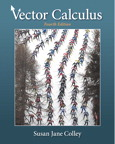• Textbook. The text for the course is Vector Calculus, fourth edition, by Susan J. Colley, 2012. http://www.pearsonhighered.com/educator/product/Vector-Calculus/9780321780652.page
This is an excellent text. It includes a lot of extra material that you will find useful in your further studies. Keep it after the course as a good reference book.

Syllabus

Not all of the topics listed below will be covered in the same depth. Some are fundamental and will be covered in detail; others indicate further directions of study and will be treated as surveys. The only concepts of physics we will study in depth are velocity, acceleration, angular velocity, and angular acceleration, but a few others will be mentioned such as force.

There are probably more topics than we can discuss in one semester, so some will have to be eliminated because of time. Likely candidates are those in brackets.

The listed exercises are tentative. They may be changed as the course progresses.

1. Vectors       4 meetings

Much of chapter 1 is a review of material you’ve already seen in Math 122 or in Math 130, but some of it will be new.

§ 1.1   Vectors in two and three dimensions
• R2, R3, vector notation, scalars
• Vector addition, zero vector, scalar multiplication, and their properties
• Geometric interpretation of vectors, parallelogram law for addition
• Exercises: 1–11 odd, 15, 23, 25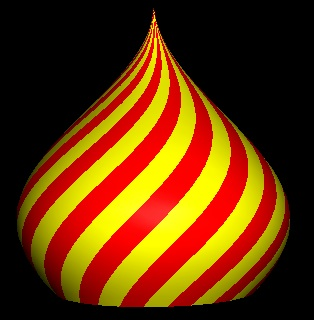§ 1.2   Vectors and equations
• Standard basis vectors i, j, k
• Parametric equations for lines
• Symmetric form equations for a line in R3
• Parametric equations for curves x :  R → R2
• Velocity, speed, and acceleration
• Exercises: 1–7 odd, 13, 15, 17, 35, 44

§ 1.3   Dot products
• Dot products of vectors, lengths of vectors, law of cosines and angles
• Projections of vectors
• Normalization of vectors
• Exercises: 1–13 odd, 17, 21, 29, 30

§ 1.4   Cross products
• Cross products of pairs of vectors in R3
• Areas of parallelograms and triangles
• Matrices, determinants
• Triple scalar product, volume of a parallelepiped
• Rotation, angular velocity
• Exercises: 1, 3, 5, 11–19 odd, 25

§ 1.5   Equations and distance
• Equations of planes, parametric equations of planes
• Distance between a point and a line
• Distance between parallel planes
• Distance between skew lines
• Exercises: 1, 3, 5, 13, 23, 25, 27, 31

§ 1.6   Summary of geometry in Rn
• Cauchy inequality, triangle inequality
• Standard basis
• Linear functions correspond to matrices, and composition of functions corresponds to matrix multiplication
• Hyperplanes
• Determinants, minors, and cofactors
• Exercises: 3, 5, 7, 16–19, 21–23, 25, 27, 28a, 30a

§ 1.7   Coordinate systems
• Rectangular coordinates
• Polar coordinates for planes
• Cylindrical and spherical coordinates for space
• Exercises: 1–8, 11, 12, 15–17

2. Differentiation in several variables       8 meetings

You’ll see how concepts of limit, continuity, and derivatives generalize from the one-variable case you saw in first-year calculus to many variables.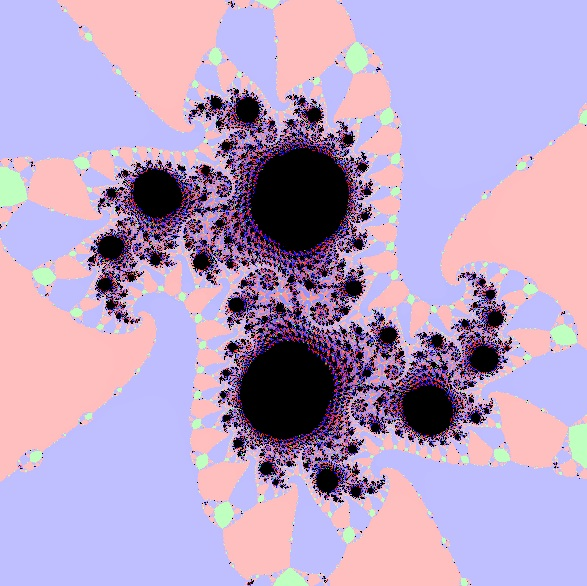§ 2.1   Functions of several variables
• Functions f :  X → Y, domain X, codomain Y, range R(f)
• Onto (surjective and one-to-one (injective) functions
• One-to-one correspondences (bijective functions) and their inverses
• Scalar-valued functions f :  Rn → R, also called scalar fields
• Vector-valued functions f : Rn → Rm, and their component functions fi : R → Rm
• Graphs of functions R2 → R as surfaces in R3, and their level curves
• Surfaces in R3, hypersurfaces in Rn
• Exercises: 1–7, 10, 15–21 odd, 31, 39

§ 2.2   Limits of vector-valued functions
• Intuitive concept and formal definition of limits for multivariate functions
• Topological concepts:  open and closed subsets, boundaries of subsets, neighborhoods of points
• Properties of limits
• Multivariate polynomials
• Continuous functions
• Exercises: 7–13, 29, 30, 38–43, 47, 48

§ 2.3   Derivatives for multivariate functions
• Partial derivatives for scalar-valued functions (scalar fields)
• Differentiability and planes tangent to surface graphs of functions R2 → R
• Differentiability and hyperplanes tangent to hypersurface graphs of functions Rn → R
• Differentiability for vector-valued functions
• Gradient vectors for scalar-valued functions
• Derivative matrix for vector-valued functions
• Exercises: 1–7, 12–14, 19–21, 29, 30, 34–36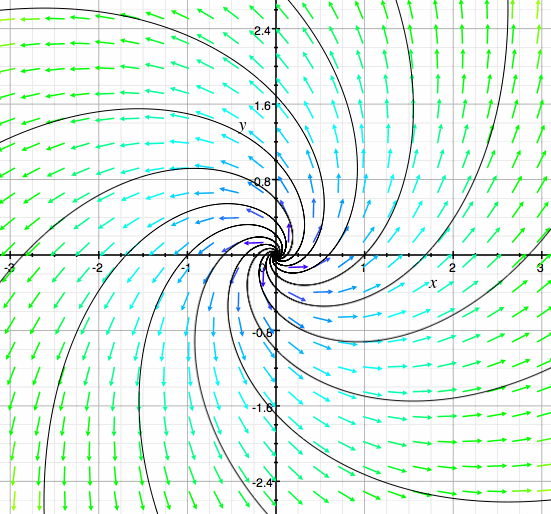§ 2.4   Properties of derivatives, higher-order partial derivatives
• Linearity:  sum, difference, and constant multiple rules for vector-valued functions Rn → Rm
• Product and quotient rules for scalar-valued functions Rn → R
• Partial derivatives of higher order
• Exercises: 1, 2, 9–11, 20, 28, 29a

§ 2.5   The chain rule in several variables
• The chain rule for composition fog where g : R → Rn and f : Rn → R
• The chain rule for the composition fog where g : Rn → Rm and f : Rm → Rp
• Polar-rectangular conversions
• Exercises: 1, 2, 5, 8, 11, 15, 19, 22, 23

§ 2.6   Directional derivatives and gradients, implicit & inverse function theorems
• The gradient vector field for a scalar field
• Directional derivatives, definition and evaluation in terms of the gradient
• Steepest ascent
• Tangent planes and hyperplanes
• Exercises: 2, 3, 12, 13, 15, 16

§ 2.7   [Newton’s method]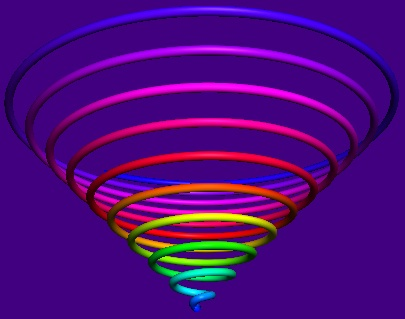3. Vector-valued functions       5 meetings

§ 3.1   Parameterized curves; Kepler’s laws of planetary motion
• Functions x : R → Rn as paths or parameterized curves
• Velocity, speed, and acceleration
• Kepler’s laws of planetary motion
• Exercises: 1–4, 7, 8, 15, 16

§ 3.2   Arc length, curvature
• Length of a path as an integral
• Unit tangent vector, curvature of a path or curve
• Exercises: 1, 2, 4, 10, 16, 22a

§ 3.3   Vector fields
• Functions f : Rn → R as scalar fields
• Functions F : Rn → Rn as vector fields
• The gradient field (a vector field) associated to a potential function (a scalar field)
• Equipotential sets
• Flow lines of vector fields
• Exercises: 1, 4, 9, 10, 19–21, 24, 26

§ 3.4   Gradient, divergence, curl, the del operator
• The del operators and gradients
• The del operator and divergence of a vector field
• The curl of a vector field in R3
• Div(curl F) = 0
• Exercises: 1–4, 7–10, 13, 28a

4. Maxima and minima in several variables       4 meetings

§ 4.1   Differentials and Taylor’s theorem
• Taylor’s theorem for single variable functions as an extension of the mean value theorem
• Taylor polynomials, remainder term
• The first-order formula for the multivariate Taylor’s theorem
• Total differentials
• The second-order formula and the Hessian
• Higher-order Taylor polynomials
• Exercises: 1, 2, 8, 9, 11, 19, 20, 24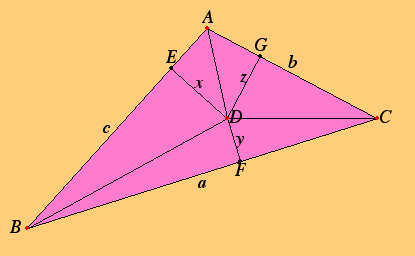§ 4.2   Maxima and minima (extrema) of functions
• Local minima and maxima for scalar fields
• Critical points and the Hessian criterion
• Quadratic forms, positive definite forms
• The second derivative test for extrema of scalar-valued functions
• Compact sets, the Extreme Value Theorem (EVT)
• Exercises: 3–6, 13–16

§ 4.3   Lagrange multipliers
• Equational constraints
• Lagrange multipliers for extrema subject to constraints
• Exercises: 3, 4, 5, 9

§ 4.4   Applications of extrema
• [Least squares approximation]
• [Applications to economics]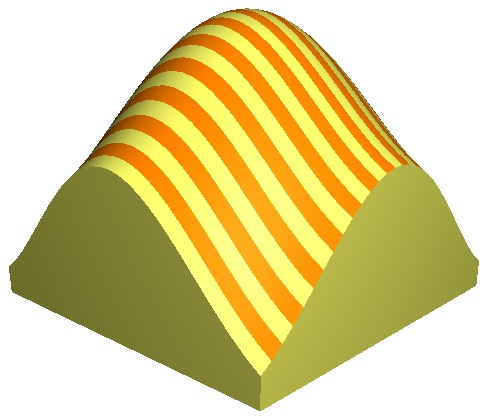5. Multiple integration       6 meetings

§ 5.1   Areas and volumes
• Volumes over rectangles as double integrals
• Exercises: 1, 2, 3, 6, 9

§ 5.2   Double integrals
• Double integrals over rectangles defined as Riemann sums, integrability
• Conditions for integrability
• Fubini’s theorem, linearity of integrals, other basic properties
• Double integrals over general regions
• Exercises: 5–7, 10, 16

§ 5.3   Changing order of integration
• Exercises: 3–6, 15, 17§ 5.4   Triple integrals
• Triple integrals over boxes
• Properties of triple integrals
• Triple integrals over general regions
• Exercises: 1, 2, 5, 6, 11, 13, 17

§ 5.5   Change of variables
• Transformations of the plane R2 → R2
• Linear transformations and their expansion factors
• Change of variables in definite integrals of one variable
• Change of variables for double integrals, the Jacobian
• Double integrals in polar coordinates
• Change of variables for triple integrals
• Exercises: 1, 3, 9, 13, 17

§ 5.6   Applications of multiple integration
• [Mean value (average value) of a scalar-valued function]
• [Center of gravity]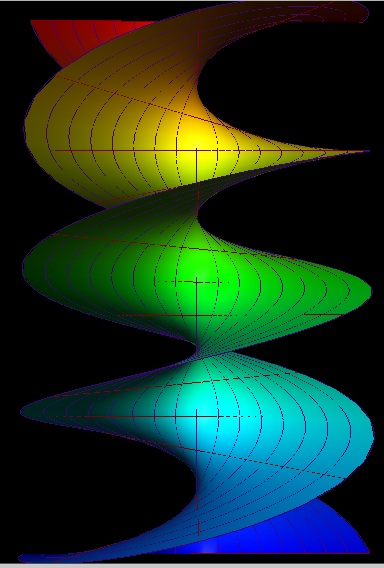6. Line integrals       5 meetings

§ 6.1   Scalar and vector line integrals
• Scalar line integrals
• Vector line integrals
• Reparameterization
• Exercises: 1–3, 9, 16, 17, 34

§ 6.2   Green’s theorem
• Green’s theorem
• Divergence theorem in the plane
• Exercises: 1–3, 7, 9, 15, 17

§ 6.3   Conservative vector fields
• Vector fields with path-independent line integrals
• Gradient fields and line integrals, conservative vector fields
• Exercises: 3–6

7. Surface integrals       5 meetings

§ 7.1   Parameterized surfaces
• Coordinate curves, normal vectors, tangent planes
• Smooth and piecewise smooth surfaces
• Areas of surfaces
• Exercises: 1, 3, 24, 26

§ 7.2   Surface integrals
• Scalar surface integrals
• Vector surface integrals
• Reparameterization of surfaces
• Exercises: 1, 3, 7, 11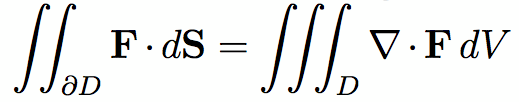§ 7.3   Stokes’ and Gauss’ theorems
• Stokes’ theorem
• Gauss’ theorem
• Divergence and curl
• Exercises: 1, 3, 7, 9
Class notes, quizzes, tests, homework assignments

The dates for the discussion topics and the assignments are tentative. They will change as the course progresses.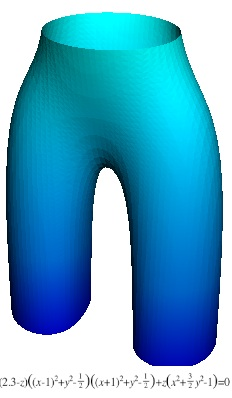1. Monday, 13 Jan 2014. Welcome to the class! Outline of the course.
Things you need to know about linear algebra
Preview. We’ll study functions Rn → Rm where not both n and m are 1. Three important kinds of these.
• When m = 1, f :  Rn → R, is a scalar-valued function or a scalar field.
• When n = 1, x : R → Rn parameterizes a curve in n-space, that is, the path of a moving point.
• When m = n, F : Rn → Rn describes a vector field on Rn.

2. Wednesday, 15 Jan.
Notes on Curves and paths. Velocity, speed, and acceleration
Quick review of important topics in linear algebra.
Discuss exercises from sections 1.1: Exercises: 1–11 odd, 15, 23, 25, and 1.2. Exercises: 1–7 odd, 13, 15, 17, 35, 44.

3. Friday, 17 Jan. More topics from linear algebra including dot products and cross products.
Dot and cross products
Discuss exercises from sections 1.3: Exercises: 1–13 odd, 17, 21, 29, 30.

Review Sets and set notation when we have time.

Monday, 20 Jan. Martin Luther King day. No classes.

4. Wednesday, 22 Jan. Discuss functions of several variables including concepts of domain, codomain, range; onto (surjective) and one-to-one (injective) functions, one-to-one correspondences (bijective functions) and their inverses; scalar-valued functions (also called scalar fields), vector-valued functions and their component functions.
Notes on Functions.
Homework due from sections 1.4: Exercises: 1, 3, 5, 11–19 odd, 25.
Discuss section 1.5: Exercises: 1, 3, 5, 13, 23, 25, 27, 31.
5. Friday, 24 Jan.
Graphs of functions as surfaces and described by level curves; surfaces and hypersurfaces
Limits of functions of several variables including the intuitive concept and formal definition of limits for multivariate functions; topological concepts of open and closed subsets, boundaries of subsets, neighborhoods of points
A gallery of surfaces
Notes on Limits.
Homework due from sections 1.5 (see above).
Discuss section 1.6: Exercises: 3, 5, 7, 16–19, 21–23, 25, 27, 28a, 30a.

6. Monday, 27 Jan.
Properties of limits; multivariate polynomials; continuous functions.
Partial derivatives
Notes on Partial derivatives
Exercises due from section 1.6 (see above).

7. Wednesday, 29 Jan.
Tangent planes, total derivatives, gradients for scalar fields Rn → R
Exercises due from section 2.1: 1–7, 10, 15–21 odd, 31, 39.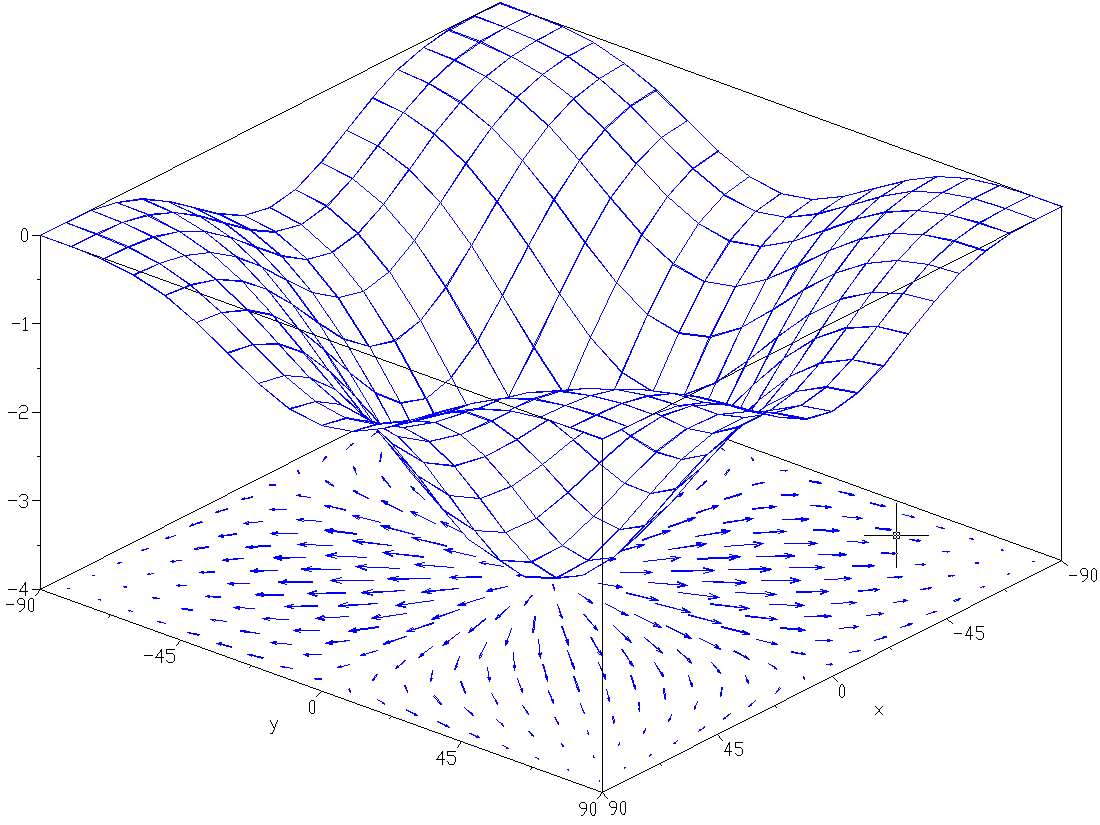8. Friday, 31 Jan.
Notes on total derivatives
Derivative matrix for vector-valued functions Rn → Rm, rules of differentiation
Exercises due from section 2.2: 7–13, 29, 30, 38–43, 47, 48.

9. Monday, 3 Feb.
Quiz on dot and cross products. Answers
Higher order derivatives
Discuss Exercises from section 2.3: 1–10, 15–17, 21, 22, 26–28 due Wed.

10. Wednesday, 5 Feb. Snow day. No classes.

11. Friday, 7 Feb.
Newton’s method
Chain rule, part 1
Exercises from section 2.3 due, see above.
Discuss Exercises due from section 2.4: 1, 2, 9–11, 16, 20, 21a due Monday.

12. Monday, 10 Feb.
Chain rule, part 2
Exercises from section 2.4 due, see above.
Discuss Exercises from section 2.5: 1, 2, 5, 8, 9, 11, 15–17
13. Wednesday, 12 Feb.
Directional derivatives, steepest ascent, tangent planes
Exercises from section 2.5 due, see above.
Discuss Exercises due Friday from section 2.6: 2, 3, 12, 13, 15, 16

14. Friday, 14 Feb.
Speed, velocity, acceleration
Exercises from section 2.6 due, see above.

15. Monday, 17 Feb. Review.
Discuss Exercises from section 3.1: 1–4, 7, 8, 15, 16 due Monday

16. Wednesday, 19 Feb. First midterm on chapter 2.

17. Friday, 21 Feb.
Arclength, the arclength parameter, and parameterizing curves by their arclength
Kepler’s laws
The arc length parameter s

18. Monday, 24 Feb.
The unit tangent vector and curvature
Exercises from section 3.1: due, see above

19. Wednesday, 26 Feb.
Vector fields, gradient fields, potential functions, equipotential sets, flow lines
Notes on Vector fields
Exercises due from section 3.2: 1, 2, 4, 8, 12, 18a.

20. Friday, 28 Feb.
Critical points, maxes, mins, saddle points,
Notes on Critical points
Exercises due from section 3.3: 1, 4, 9, 10, 19–21, 24, 26.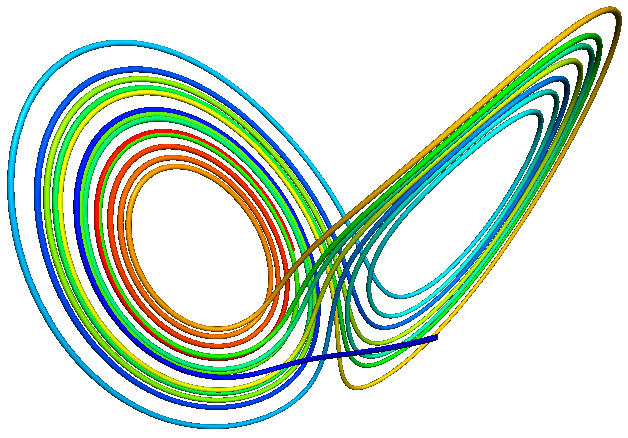Monday–Friday, 3–7 Mar. Spring Break

21. Monday, 10 Mar.
The del operator, divergence of vector fields, incompressible vector fields, curl of a vector field, irrotational vector fields
Notes on Gradient, divergence, and curl
A Lorenz attractor
Discuss Exercises due Wednesday from section 3.4: 1--4, 7--10, 13, 28a.

22. Wednesday, 12 Mar.
Taylor's theorem for a single variable, Taylor polynomials, remainder term; the first-order formula for the multivariate Taylor's theorem, total differentials; the second-order formula and the Hessian.
Notes on Differentials and Taylor polynomials
Exercises from section 3.4 due, see above
Discuss Exercises due Friday from section 4.1: 1, 2, 8, 9, 11, 19, 20, 24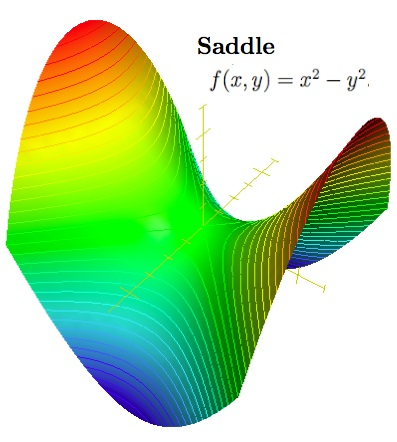23. Friday, 14 Mar.
Local minima and maxima for scalar fields, critical points and the Hessian criterion; quadratic forms, positive definite form; the second derivative test
Compact sets, the Extreme Value Theorem (EVT)
Maxima and minima of scalar fields
Exercises from section 4.1 due, see above.

24. Monday, 17 Mar.
Quiz on sections 3.2–3.4.
Discuss Exercises due Wednesday from section 4.2: 3--6, 13--16
Lagrange multipliers

25. Wednesday, 19 Mar.
An application of Lagrange multipliers to pyramids
Exercises from section 4.2 due, see above.
Discuss Exercises due Friday from section 4.3: 3, 4, 5, 9.

26. Friday, 21 Mar.
Double integrals. Volumes as integrals, integration over rectangles and other regions, Riemann sums, integrability.
Exercises due from section 4.3 due, see above.
Discuss Exercises due Monday from section 5.1 and 5.2

27. Monday, 24 Mar.
Fubini’s theorem, linearity of integrals, other basic properties. Double integrals over general regions. Changing order of integration. Triple integrals over boxes.
Exercises due from section 5.1: 1, 2, 3, 6, 9,
and from section 5.2: 5, 6, 7, 10, 16.

28. Wednesday, 26 Mar.
Jacobians for change of variables. Transformations of the plane R2 → R2 and their expansion factors. Change of variables and the Jacobian for double and triple integrals
Double integrals in polar coordinates
Exercises due from section 5.3: 3–6, 15, 17,

29. Friday, 28 Mar.
Exercises due from section 5.4: 1, 2, 5, 6, 11, 13, 17

30. Monday, 31 Mar. Review.

31. Wednesday, 2 Apr. Second midterm on chapters 3–5.

32. Friday, 4 Apr.
Introduction to line integrals: scalar line integrals, vector line integrals, and differential forms.
Line integrals
33. Monday, 7 Apr.
Green's theorem as a generalization of the fundamental theorem of calculus, Stokes' theorem and the divergence theorem in the plane.
Green’s theorem

34. Wednesday, 9 Apr. Spree day. No classes.

35. Friday, 11 Apr.
Proof of Green’s theorem
Exercises from section 5.5: 1, 3, 9, 13, 17 due

36. Monday, 14 Apr.
Irrotational vector fields with simply connected domains are conservative.
Exercises from section 6.1.: 1–3, 9, 16, 17, 34 due

37. Wednesday, 16 Apr.
Conservative vector fields: as gradient fields, as vector fields having path independent line integrals, as fields with 0 integrals over closed paths.
Exercises from section 6.2: 1–3, 7, 9, 15, 17 due.

38. Friday, 18 Apr.
Surfaces in space, their parameterizations and tangent planes.
Exercises from section 6.3: 3–6 due.

39. Monday, 21 Apr.
Scalar surface integrals
Vector surface integrals
Exercises from section 7.1: 1, 3, 24, 26 due.

40. Wednesday, 23 Apr. Academic Spree Day.
More on Stokes' theorem, orientation of surfaces, the statement of the divergence theorem, a.k.a. Gauss's theorem.
Exercises from section 7.2: 1, 3, 7, 11 due.

41. Friday, 25 Apr.
Gauss’s theorem
Exercises from section 7.3: 1, 3, 7, 9 due Monday.

42. Monday, 28 Apr. Review

43. Wednesday, 30 Apr. Reading day. Review

44. Tuesday, 6 May. Final Exam. 8:00–10:00.
Past tests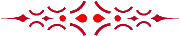`http://aleph0.clarku.edu/~djoyce/ma131/`